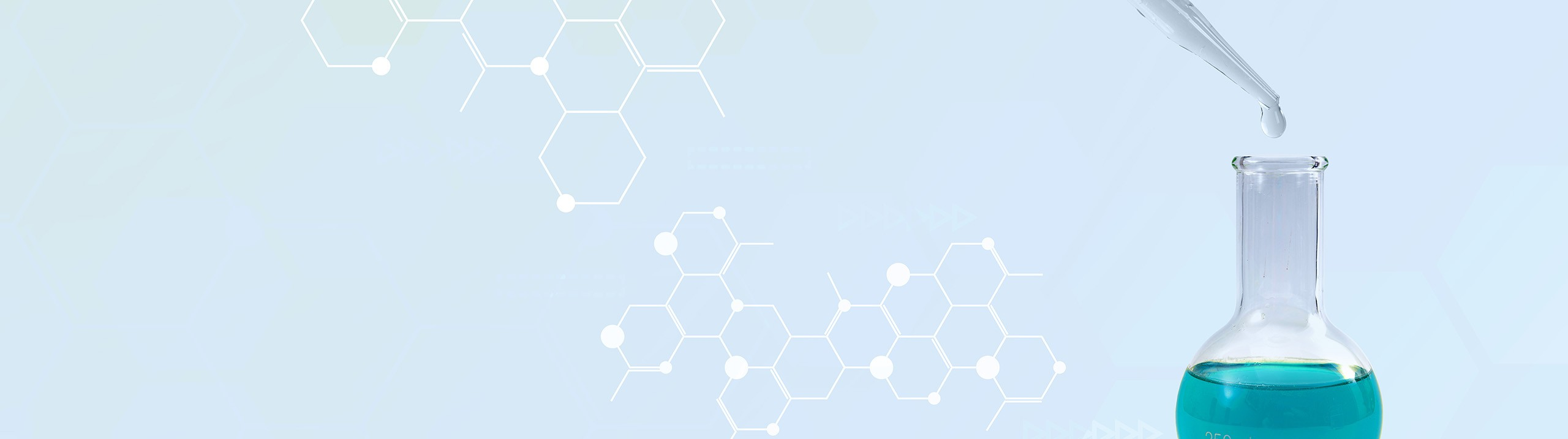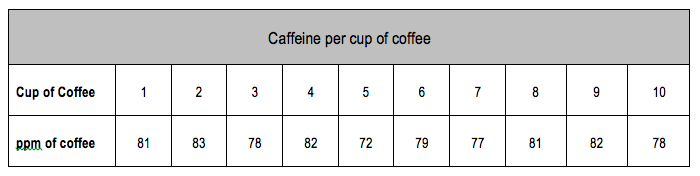Start typing, then use the up and down arrows to select an option from the list.# Analytical Chemistry

Learn the toughest concepts covered in your Analytical Chemistry class with step-by-step video tutorials and practice problems.

Statistics, Quality Assurance and Calibration Methods

When dealing with data sets it becomes important to eliminate outliers in order to have the most accurate standard deviation.

## Grubbs Test vs. Q Test

1

#### concept

Grubbs Test vs. Q Test3m
Play a video:
2

#### example

Q Test4m
Play a video:
So here it says wishing to measure the amount of caffeine in a cup of coffee. You pour 10 cups from the data provided, perform a Q. Test to determine if the outlier can be retained or disregarded. All right. So what we need to do here is we need to organize this list of measurements from smallest to largest. Alright, So We can see that the smallest number is 72. Then the next smallest number looks like it is 77, then 78 Then 78. Again than 79. Than 81 81 again, 82 twice And then finally 83. So, I've organized it from smallest measurement, two largest measurement. Remember that's important because that will give us the range that we need. Alright, so now we're gonna figure out our Q. Calculated. Remember our Q calculated will be the outlier that we're investigating minus the number that's closest to it in absolute brackets divided by our range. So remember that's gap divided by range. So actually may rewrite this. So, if you calculated his gap divided by range. Alright, So the number that we're looking at is the outlier, The one that's farthest from everyone else. So let's see that the difference between these two is five. And then we're gonna say the difference between these two is one. These are zero, this is one, This here is zero. The difference between these two is 2. The difference between these two is one. Difference between these 20 difference between these two is one. So the outlier is the one that's farthest from all the other measurements and we can see that that would be 72 72 is the farthest from everyone else. It's difference is five away from 77. So that's the outlier that we're investigating. So that's 72 Minour the value that's closest to it which is 77 Divided by the range. Remember the range is your largest value minus your smallest value. So your largest value is 83 minus your smallest value which is 72. Okay so that's gonna come out to being .455. Now if you take a look at the queue table that we have on the previous page let's say we want to look at it in terms of our 99% confidence interval. We want to have 99% confidence if this should be kept or not. Alright. So looking at the number of measures measurements we have we have 10 measurements. So looking at that table, We're looking at 10 measurements and scroll all the way to the right. Till you get to the 99 confidence value There were going to see that cute table or q critical equals .568. So comparing our Q. Calculated to our queue table, what can we say? Well we're gonna say that are cute table is a larger value than our Q. Calculated. Therefore you have to retain the value. So that number of 72 we're gonna keep it around from our 99 confidence that we were able to find out from our queue table. So that's all we have to do in order to figure out our Q. calculated. And then compared to our queue table now that you've seen this one, look to see if you can figure out the example to that's on the bottom of the page. Don't worry if you get stuck, just come back and see how I approach that same example question. And just remember some of the techniques we used here for the Q Test.3

#### example

Grubbs Test5m
Play a video: